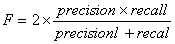SHREC 2018 - 2D Scene Image-Based 3D Scene Retrieval

Evaluation Methods

The procedure of information retrieval evaluation is straightforward. In response to a given set of users' queries, an algorithm searches the benchmark database and returns an ordered list of responses called the ranked list(s), the evaluation of the algorithm then is transformed to the evaluation of the quality of the ranked list(s).  Next, we will discuss the evaluation method.

Different evaluation metrics measure different aspects of shape retrieval behavior. In order to make a thorough evaluation of a 2D scene image-based 3D scene retrieval algorithm with high confidence, we employ a number of common evaluation measures used in the information retrieval community.

Precision- Recall

Precision- Recall Graph is the most common metric to evaluate information retrieval system. Precision is the ratio of retrieved objects that are relevant to all retrieved objects in the ranked list. Recall is the ratio of relevant objects retrieved in the ranked list to all relevant objects.

Let A be the set of all relevant objects, and B be the set of all retrieved object then,,(1)

Basically, Recall evaluates how well a retrieval algorithm finds what we want and precision evaluates how well it weeds out what we don't want. There is a tradeoff between Recall and Precision, one can increase Recall by retrieving more, while, this can decrease Precision.

E-Measures

The idea is to combine precision and recall of into a single number to evaluation the whole system performance. First we introduce the F-measure, which is the weighted harmonic mean of precision and recall. F-measure is defined as, whereis the weight.                         (2)

Letbe 1, the weight of precision and recall is same, and we have(3)

Then, go over all points on the precision-recall curve of each model and compute the F-measure, we get the overall evaluation of F for a special algorithm.

The E-Measure is defined as E = 1- F,(4)

Note that the maximum value is 1.0, and higher values indicate better results. The fact is that a user of a search engine is more interested in the first page of query results than in later pages. So, here we consider only the first 32 retrieved objects for every query and calculates the E-Measure over those results.

Cumulated gain -based measurements

Based on the idea that the greater the ranked position of a relevant object the less valuable it is for the user, because the less likely it is that the user will examine the object due to time, effort, and cumulated information from objects already seen.

In this evaluation, the relevance level of each object is used as a gained value measures for its ranked position m, the result and the gain is summed progressively from position 1 to n. Thus the ranked object lists (of some determined length) are turned to gained value lists by replacing object IDs with their relevance values. The binary relevance values 0, 1 are used (1 denoting relevant, 0 irrelevant) in our benchmark evaluation.  Replace the object ID with the relevance values, we have for example:

G'=< 1, 1, 1, 0, 0, 1, 1,0,1,0 . . . . >

The cumulated gain at ranked position i is computed by summing from position 1 to i when i ranges from 1 to the length of the ranking list. Formally, let us denote position i in the gain vector G by G[i]. The cumulated gain vector CG is defined recursively as the vector CG where:(5)

The comparison of matching algorithms is then equal to compare the cumulated gain, the greater the rank, the smaller share of the object value is added to the cumulated gain. A discounting function is needed which progressively reduces the object weight as its rank increases but not too steeply:(6)

The actual CG and DCG vectors by a particular matching algorithm may also be compared to the theoretically best possible. And this is called normalized CG, normalized DCG. The latter vectors are constructed as follows. Let there be 5 relevant objects, and 5 irrelevant objects in each class, then, at the relevance levels 0 and 1. Then the ideal  Gain vector is  obtain by filling the first vector positions with 1, and  the remaining positions by the values 0. Then compute CG and DCG as well as the average CG and DCG vectors and curves as above. Note that the curves will turn horizontal when no more relevant objects (of any level) can be found. The vertical distance between an actual DCG/CG curve and the theoretically best possible curve shows the effort wasted on less-than-perfect objects due to a particular matching algorithm.

Nearest Neighbor (NN), First-tier (Tier1) and Second-tier (Tier2)

These evaluation measures share the similar idea, that is, to check the ratio of models in the query's class that also appear within the top K matches, where K can be 1, the size of the query's class, or the double size of the query's class. Specifically, for a class with |C| members, K= 1 for Nearest Neighbor, K = |C| − 1 for the first tier, and K = 2 *(|C| − 1) for the second tier. For this evaluation C is always 20. The final score is an average over all the objects in database.